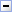# Formula Constructor (function, range, cellCompare)

#### Yogesh.ExcelXml

A Sandcastle Documented Class Library Formula Constructor (function, range, cellCompare)
NamespacesYogesh.ExcelXmlFormulaFormula(String, Range, Predicate<(Of <(Cell>)>))
Constructs a formula and adds a filtered range as the first parameterDeclaration Syntax
 C# Visual Basic Visual C++
```public Formula(
string function,
Range range,
Predicate<Cell> cellCompare
)```
```Public Sub New ( _
function As String, _
range As Range, _
cellCompare As Predicate(Of Cell) _
)```
```public:
Formula(
String^ function,
Range^ range,
Predicate<Cell^>^ cellCompare
)```Parameters
function (String)
Function name
range (Range)
cellCompare (Predicate<(Of <(Cell>)>))
A custom defined to compare the values of the rangeRemarks
Custom delegates can filter all cells and auto add them to the parameter list of a formula by passing a System.Predicate>Cell<, i.e. a delegate which accepts Cell as its value and returns bool to both Formula constructor or Add. All the values accessors (i.e. Value, NumericValue etc.) and cell style can be checked.Examples
Lets assume column 1,2,3,6 and 7 are bold...CopyC#
```XmlStyle style = new XmlStyle();
style.Font.Bold = true;

// VS2008 style
sheet[7, 3].Value = new Formula("sum", new Range(sheet[0, 3], sheet[6, 3]),
cell => cell.Style == style);

// or VS2005 style
sheet[7, 3].Value = new Formula("sum", new Range(sheet[0, 3], sheet[6, 3]),
delegate (Cell cell) { return cell.Style == style; } );```
In the first example of style, the value of the cell will be =SUM(A4:C4, F4:G4).

Continuous ranges matching to true will be joined as one parameter, i.e. A4:C4 and not as seperate parameters, i.e. A4,B4,C4

Using value accessors...CopyC#
```sheet[7, 3].Value = new Formula("sum", new Range(sheet[0, 3], sheet[6, 3]),
cell => cell.NumericValue > 10000 & cell.NumericValue <= 50000);```

Assembly: Yogesh.ExcelXml (Module: Yogesh.ExcelXml) Version: 2.89.501.2158